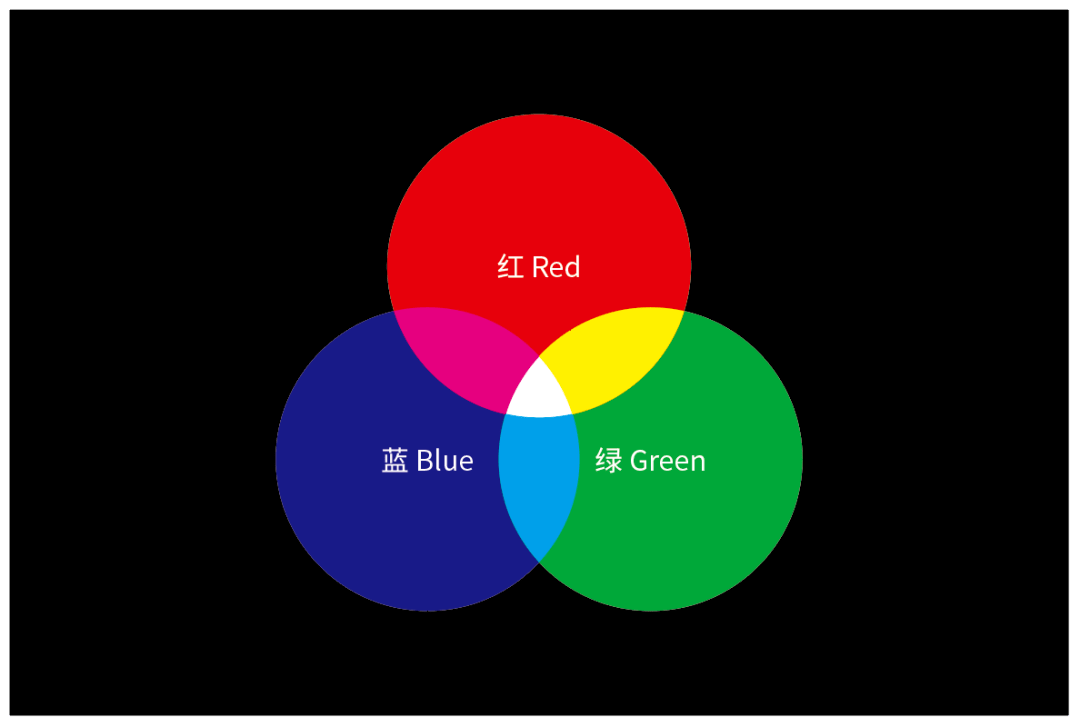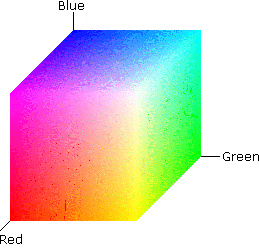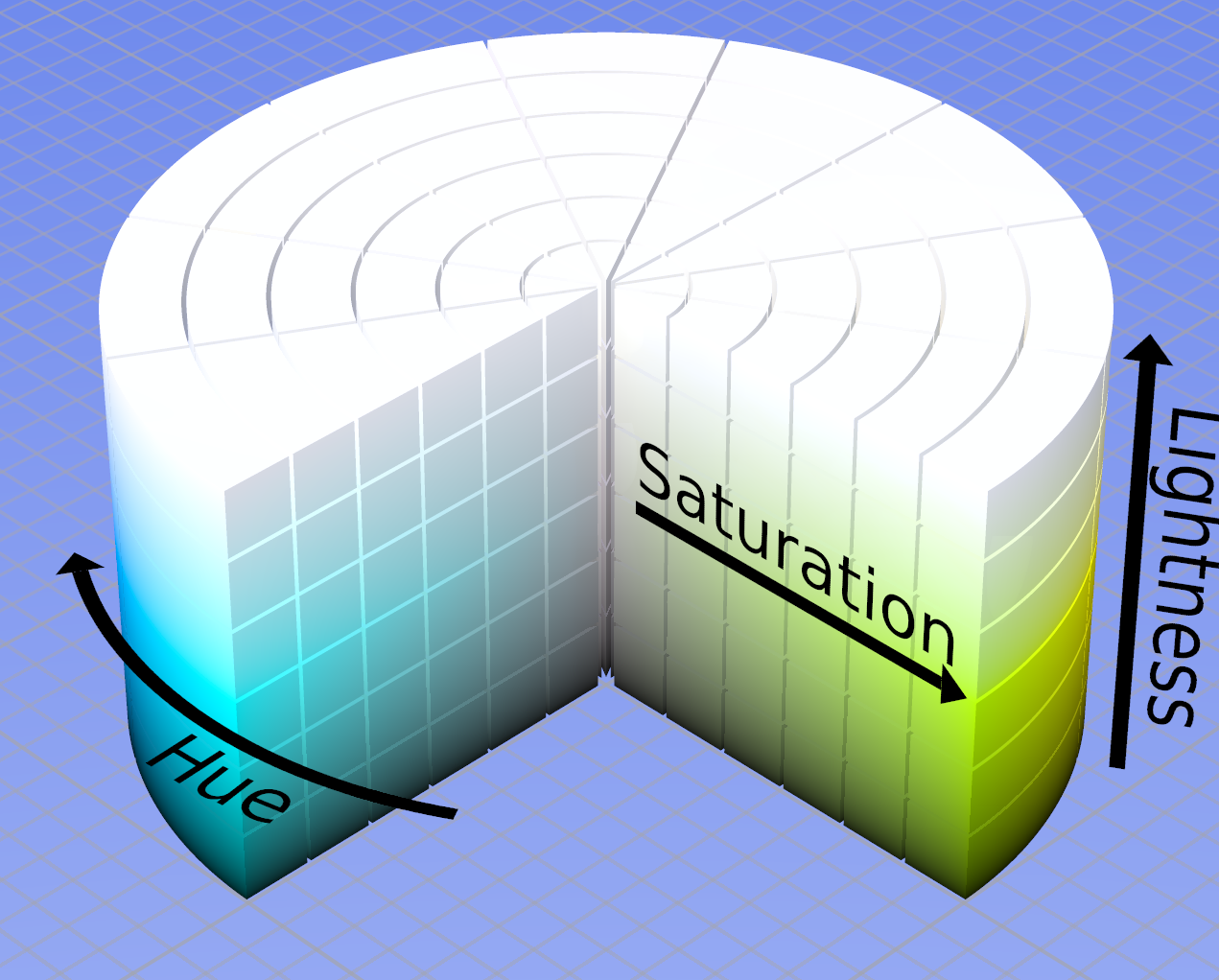# CSS 中颜色的表示和转换

## 基本概念## CSS 中颜色的表示和转换

### 方式一：颜色词

```css color: red; // 红色 color: green; // 绿色 color: blue; // 蓝色```

### 方式二：RGB

```css color: rgb(255, 0, 0, 1); // 红色 color: rgb(0, 255, 0, 1); // 绿色 color: rgb(0, 0, 255, 1); // 蓝色```

RGB 是以一组十进制数值表示颜色，结构为 `rgb(R, G, B, A)`（其中 A 表示透明度），RGB 色彩模型的创建方式是将红色、绿色、蓝色映射到三维笛卡尔坐标系中。### 方式三：HEX

```css color: #FF0000; // 红色 color: #00FF00; // 绿色 color: #0000FF; // 蓝色```

HEX 是以一组十六进制数值表示颜色，结构为 `#RRGGBB[AA]``#RGB[A]`（其中 A 表示透明度），下面这个等式可以成立：

```css color: #FF0000; // 等价于 color: #F00; // 等价于 color: #F00F;```

RGB 转 HEX 算法：

```javascript // 摘自 https://github.com/bgrins/TinyColor/blob/master/tinycolor.js#L497 function rgbToHex(r, g, b, allow3Char) { var hex = [ pad2(mathRound(r).toString(16)), pad2(mathRound(g).toString(16)), pad2(mathRound(b).toString(16)) ];

// Return a 3 character hex if possible if (allow3Char && hex.charAt(0) == hex.charAt(1) && hex.charAt(0) == hex.charAt(1) && hex.charAt(0) == hex.charAt(1) ) { return hex.charAt(0) + hex.charAt(0) + hex.charAt(0); } return hex.join(""); } ```

### 方式四：HSL

```css color: hsl(0, 1000%, 50%); // 红色 color: hsl(120, 100%, 50%); // 绿色 color: hsl(240, 100%, 50%); // 蓝色```

HSL 的结构为 `hsl(H,S,L,A)`（其中 A 表示透明度），是一种将 RGB 色彩模型中的点在圆柱坐标系中的表示方式，这种色彩模型能够做到比基于笛卡尔坐标系的几何结构更加直观。• 色相（Hue）：取值 0～360，指色彩的基本属性，0（或360）表示红色，120 表示绿色，240表示蓝色。
• 饱和度（Saturation）：取值 0～100%，指色彩的纯度，越高色彩越纯，低则逐渐变灰。
• 明度（Lightness）：取值 0～100%。

RGB 转 HSL 算法：

```javascript // 摘自 https://github.com/bgrins/TinyColor/blob/master/tinycolor.js#L379 function rgbToHsl(r, g, b) { r = bound01(r, 255); g = bound01(g, 255); b = bound01(b, 255); var max = mathMax(r, g, b), min = mathMin(r, g, b); var h, s, l = (max + min) / 2; if (max == min) { h = s = 0; // achromatic } else { var d = max - min; s = l > 0.5 ? d / (2 - max - min) : d / (max + min); switch (max) { case r: h = (g - b) / d + (g < b ? 6 : 0); break; case g: h = (b - r) / d + 2; break; case b: h = (r - g) / d + 4; break; }

``````h /= 6;
``````

} return { h: h, s: s, l: l }; } ```